Maths
Study Material

# Combination Formula – Choose k from n

Learn the approaches and tricks to solve any problem that starts with the phrase choose k objects from n.

4 minutes long
Posted by Tanwir Silar, 11/8/2021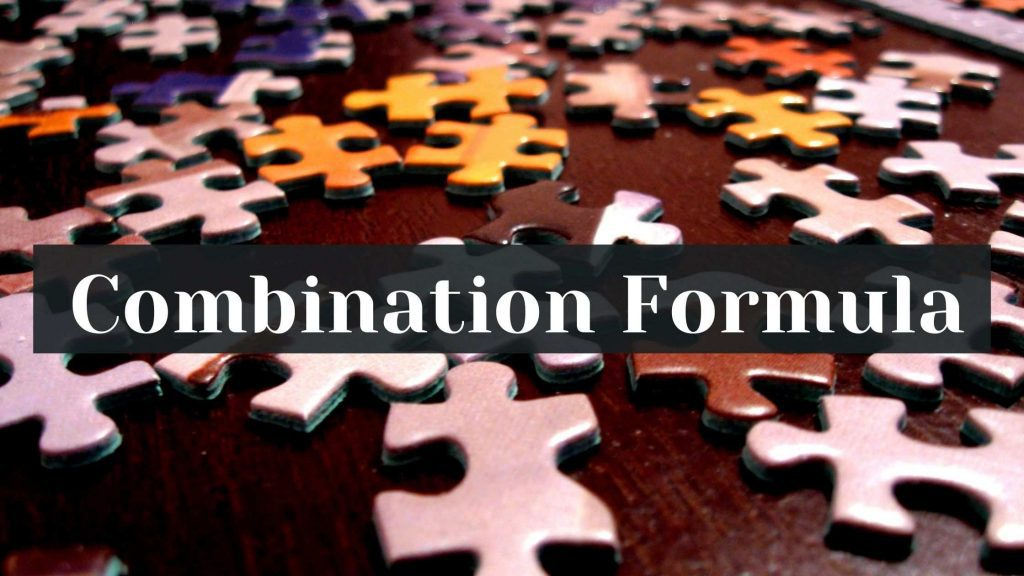Hesap Oluştur

Got stuck on homework? Get your step-by-step solutions from real tutors in minutes! 24/7. Unlimited.

Often in combinatorics, we are asked to find the number of ways to choose k objects/things from n objects/things.(Combination formula)

## Different ways of choosing k from n

There are four ways of choosing k objects/things from n objects/things. They are:

1. Choosing k objects from a group of n with replacement where order matters
2. Choosing k objects from a group of n without replacement where order matters
3. Choose k objects from a group of n without replacement when order doesn’t matter
4. Choose k objects from a group of n with replacement when order doesn’t matter.

### 1. Choosing k objects from a group of n with replacement where order matters

Label each of the objects as 1, 2, 3, … , n. Since, we choose an item and replace it afterwards, every time we need to choose an object we have n choices.
So the answer is n * n * n * … k times, equals to nk.

### 2. Choosing k objects from a group of n without replacement where order matters

Before answering this question, we need to know that the number of permutations of m distinct objects taken all at a time is m!. We also know that the number of ways of choosing k objects from a group of n is , which is also referred to as n choose k. So the answer for this would be to choose k from n ( n choose k ) and then since order matters multiply by all the permutations possible (k!). The number of ways =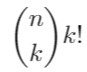### 3. Choosing k objects from a group of n without replacement and order doesn’t matter

This problem is similar to the above problem, except that order doesn’t matter here. The answer is equal to the number of ways to choose k objects from n (n choose k ),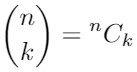.

### 4. Choosing k objects from a group of n with replacement and order doesn’t matter.

This is no ordinary problem! In order to solve this, we need to map this problem to an equivalent problem. Let there are n (distinct) boxes and k identical balls. The answer to the above (4) question is equal to the number of ways to put these k identical balls in the n distinct boxes, which we achieve by answering the equivalent problem.

Here, each of the k balls could go to any of the n boxes equally likely. The equivalence to our problem comes from the fact that we choose each box (object) number of times = the number of balls in it, in our original problem.
And the number of ways to put these k balls into the n boxes is equals to the number of ways of arranging n-1 separators between these k balls. This is equals to choosing k places out of n + k -1 places to place the balls and the remaining places go to the separators. The answer is equal to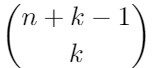.

This table below summarizes the four ways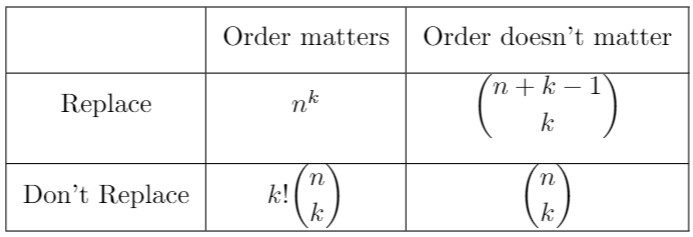We hope, our blog(combination formula) was helpful.